# Subaru Outback: Calculating total and load capacities varying seating configurations

Calculate the available load capacity as shown in the following examples:

Example 1A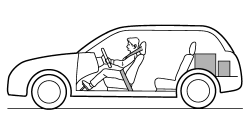Vehicle capacity weight of the vehicle is 800 lbs (363 kg), which is indicated on the vehicle placard with the statement “The combined weight of occupants and cargo should never exceed 363 kg or 800 lbs”.

For example, if the vehicle has one occupant weighing 154 lbs (70 kg) plus cargo weighing 551 lbs (250 kg).

1. Calculate the total weight.2. Calculate the available load capacity by subtracting the total weight from the vehicle capacity weight of 800 lbs (363 kg).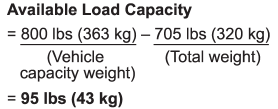3. The result of step 2 shows that a further 95 lbs (43 kg) of cargo can be carried.

Example 1B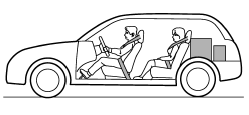For example, if a person weighing 176 lbs (80 kg) now enters the same vehicle (bringing the number of occupants to two), the calculations are as follows:

1. Calculate the total weight.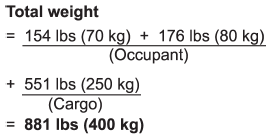2. Calculate the available load capacity.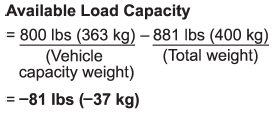3. The total weight now exceeds the capacity weight by 81 lbs (37 kg), so the cargo weight must be reduced by 81 lbs (37 kg) or more.

Example 2A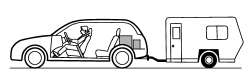Vehicle capacity weight of the vehicle is 800 lbs (363 kg), which is indicated on the vehicle placard with the statement “The combined weight of occupants and cargo should never exceed 363 kg or 800 lbs”.

For example, the vehicle has one occupant weighing 165 lbs (75 kg) plus cargo weighing 265 lbs (120 kg). In addition, the vehicle is fitted with a trailer hitch weighing 22 lbs (10 kg), to which is attached a trailer weighing 1,764 lbs (800 kg). 10% of the trailer weight is applied to the trailer tongue (i.e. Tongue load = 176 lbs (80 kg)).

1. Calculate the total weight.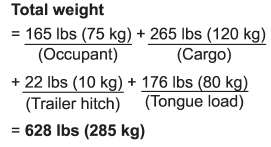2. Calculate the available load capacity.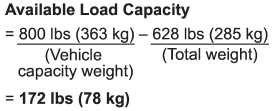3. The result of step 2 shows that a further 172 lbs (78 kg) of cargo can be carried.

Example 2B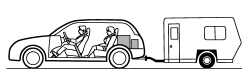For example, if a person weighing 143 lbs (65 kg) and a child weighing 40 lbs (18 kg) now enter the same vehicle (bringing the number of occupants to three), and a child restraint system weighing 11 lbs (5 kg) is installed in the vehicle for the child to use, the calculations are as follows:

1. Calculate the total weight.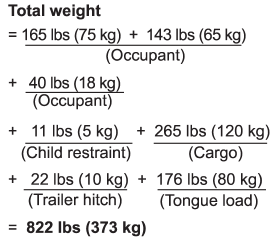2. Calculate the available load capacity.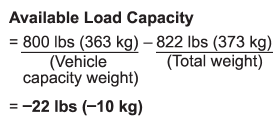3. The total weight now exceeds the capacity weight by 22 lbs (10 kg), so the cargo weight must be reduced by 22 lbs (10 kg) or more.

###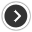Determining compatibility of tire and vehicle load capacities

The sum of four tires’ maximum load ratings must exceed the maximum loaded vehicle weight (“GVWR”). In addition, sum of the maximum load ratings of two front tires and of two rear tires must

###Adverse safety consequences of overloading on handling and stopping and on tires

Overloading could affect vehicle handling, stopping distance, vehicle and tire as shown in the following. This could lead to an accident and possibly result in severe personal injury. ● Veh

###Steps for Determining Correct Load Limit

1. Locate the statement “The combined weight of occupants and cargo should never exceed XXX pounds” on your vehicle’s placard. 2. Determine the combined weight of the driver and passengers th

## SEE MORE:

###Oil Pump

A: REMOVAL 1) Disconnect the ground cable from battery. 2) Remove the V-belts. <Ref. to ME(H4SO)-43, REMOVAL, V-belt.> 3) Remove the power steering oil pump assembly. Disconnect the connector from power steering pump switch. Disconnect the pressure hose (b) and suction hose (a) from power

###Electronic parking brake

1) Parking brake switch 2) Hill Holder switch 3) Indicator light WARNING ● Before exiting the vehicle, make sure that you turn off the engine. Otherwise, the parking brake may be released and an accident may occur. ● If the brake system warning light flashes, the electronic park